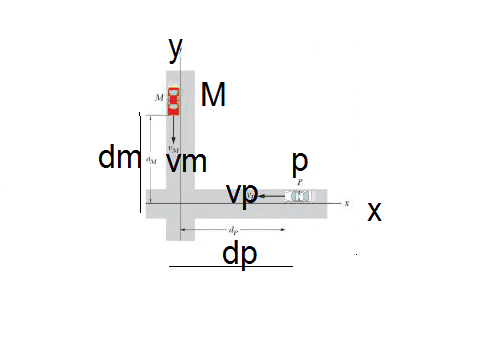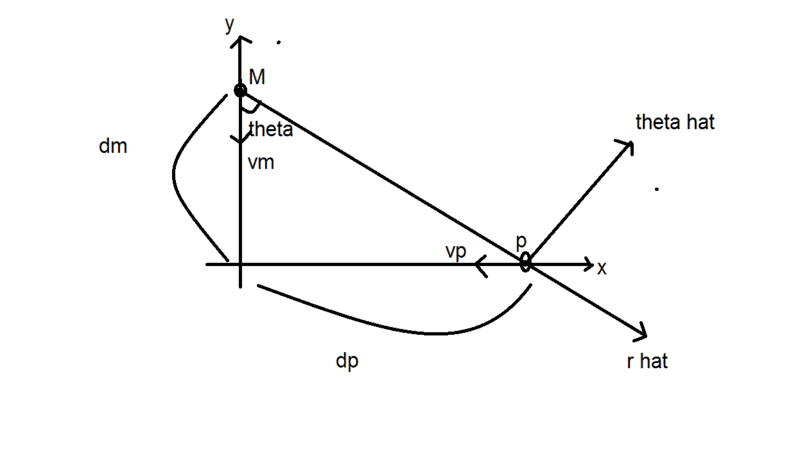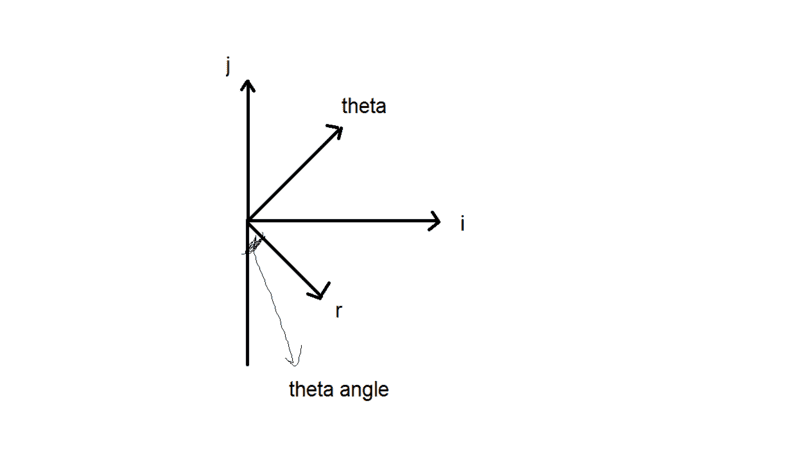# Finding the minimum distance between two cars

## Homework Statement

We have two cars moving like the picture. we want to find their minimum distance using relative motion.
I) Consider a coordinate system on M, which has two axis. one axis is in direction of MP and the other is perpendicular to that (Something like r_hat and θ_hat). Find the velocity of p in the new coordinate system.
II) Describe the movement of P from M viewpoint (I think it means find the equation of motion)
III) Using II, find minimum distance between the two cars?
IV) Prove that the minimum distance is zero if and only if dp/vp = dm/vm.
The picture is from Fundamentals of Physics/Resnick,Walker,Holiday but the question is different from the question of the book.Don't Know.

## The Attempt at a Solution

I can completely solve this without the new coordinate system and using only i and j. But I have problems with this new coordinate system. I can also find something like vp = -vp sin θ r_hat + (-vp) cos θ θ_hat. θ is the angle between MP and Y axis. But I don't know how to find the equation of motion and solve the other parts. using the method in the question.

#### Attachments

kuruman
Homework Helper
Gold Member
2021 Award
This is a relative velocity problem. Start with question (I). Assume that you are the driver of car M. What is the velocity of car P relative to you? Use ##\hat i## and ##\hat j## for your description.

This is a relative velocity problem. Start with question (I). Assume that you are the driver of car M. What is the velocity of car P relative to you? Use ##\hat i## and ##\hat j## for your description.
As I said in the the attempt at the solution part, I can solve this with i and j. But it seems the question wants it to be solved with ##\hat r## and ##\hat θ##.

kuruman
Homework Helper
Gold Member
2021 Award
Please post the statement of the problem exactly as was given to you. You mention "something like r_hat and θ_hat", but I doubt that's how it was given to you. Is there a different diagram that shows angle ##\theta##? Also, for (II) the question does not say to use ##r## and ##\theta##, so it seems you can use the Cartesian axes to answer it.

Please post the statement of the problem exactly as was given to you. You mention "something like r_hat and θ_hat", but I doubt that's how it was given to you. Is there a different diagram that shows angle ##\theta##? Also, for (II) the question does not say to use ##r## and ##\theta##, so it seems you can use the Cartesian axes to answer it.

Is is because the University teacher, showed this picture in holiday physics and then asked the question orally and said use something like r_hat and θ_hat (as a hint). So it is unknown to me that where he get the question and what is the exact statement. Usually, Books don't force anyone to use just one approach to solve a question but in this case, the question giver wants it to be solved only with ##r## and ##\theta##.

I think this picture, describe the question coordinates better: (theta hat and r hat are perpendicular)#### Attachments

kuruman
Homework Helper
Gold Member
2021 Award
That's good.
1. Express ##\vec v_{rel}## in terms of ##\hat i## and ##\hat j##.
2. Draw a new drawing, based on the one above, showing the four unit vectors ##\hat i##, ##\hat j##, ##\hat r## and ##\hat \theta##.
3. Find the coefficients ##a_{kl}## such that ##\hat i=a_{11}~\hat r+a_{12}~\hat\theta## and ##\hat j=a_{21}~\hat r+a_{22}~\hat\theta##. They should be sines and cosines involving ##\theta.##
4. Substitute in 1.

That's good.
1. Express ##\vec v_{rel}## in terms of ##\hat i## and ##\hat j##.
2. Draw a new drawing, based on the one above, showing the four unit vectors ##\hat i##, ##\hat j##, ##\hat r## and ##\hat \theta##.
3. Find the coefficients ##a_{kl}## such that ##\hat i=a_{11}~\hat r+a_{12}~\hat\theta## and ##\hat j=a_{21}~\hat r+a_{22}~\hat\theta##. They should be sines and cosines involving ##\theta.##
4. Substitute in 1.
I think ##\vec v_{rel}## = -vp ##\hat i## + vm ##\hat j##. Also ##\hat i = - cos \theta \hat r + sin \theta \hat \theta## and ##\hat j = sin \theta \hat r + cos \theta \hat \theta##

and after substitution we get an equation for ##\vec v_{rel}## in term of ##\hat r , \hat \theta ,sin ,cos## of## \theta ,vm,vp ##. Now, How should I write equation of motion? because the theta itself is not constant and change with time (as the get closer).

Last edited:
kuruman
Homework Helper
Gold Member
2021 Award
Please show your drawing of the four unit vectors. I am not sure your answers have the correct signs. Also, remember that ##\vec v_{rel} ## is the velocity of P relative to M. If P were not moving (## v_P = 0##), driver M should see P moving in the ##\hat j## direction. Your expression does not predict that.

Please show your drawing of the four unit vectors. I am not sure your answers have the correct signs. Also, remember that ##\vec v_{rel} ## is the velocity of P relative to M. If P were not moving (## v_P = 0##), driver M should see P moving in the ##\hat j## direction. Your expression does not predict that.
Yes. The ##v_{rel}## part was a typo. But in the i and j part, I think I used j instead of i. so they are actually ##\hat j = - cos \theta \hat r + sin \theta \hat \theta## and ##\hat i = sin \theta \hat r + cos \theta \hat \theta## and ##\vec v_{rel}## = -vp ##\hat i## + vm ##\hat j###### Attachments

kuruman
Homework Helper
Gold Member
2021 Award
OK. Now find ##\vec v_{rel}## in terms of ##\hat r## and ##\hat \theta.##

So ##\vec v_{rel} = -(v_p sin \theta +v_m cos \theta) \hat r + (-v_p cos\theta + v_m sin\theta)\hat \theta##. I don't know what to do with this to write equation of motion. Because ##\hat r , \hat \theta## are not constant and they also change with ##\theta##.

kuruman
Homework Helper
Gold Member
2021 Award
So ##\vec v_{rel} = -(v_p sin \theta +v_m cos \theta) \hat r + (-v_p cos\theta + v_m sin\theta)\hat \theta##. I don't know what to do with this to write equation of motion. Because ##\hat r , \hat \theta## are not constant and they also change with ##\theta##.
Right, but you can always write the position of P relative to M in Cartesian coordinates (##\vec r = \vec r_0 +\vec v_{rel}~t,## then just rewrite the Cartesian unit vectors in terms of the polar unit vectors.

Right, but you can always write the position of P relative to M in Cartesian coordinates (##\vec r = \vec r_0 +\vec v_{rel}~t,## then just rewrite the Cartesian unit vectors in terms of the polar unit vectors.
Yes I can rewrite every cartesian coordinates in terms of polar coordinates. But how I must differentiate the ##\vec r## in polar coordinates to find the minimum distance? I know the principle of differentiation of polar vectors like ##\dot \theta \ddot \theta## and others but the problem is that in this case, ##\theta## will be a ##arctan## function in terms of ##t## and differentiating the whole equation will result a very big equation (I think), and then solving the ##\dot r=0## will be more difficult. I want to know is there any technique to not calculate the very big derivative?

kuruman